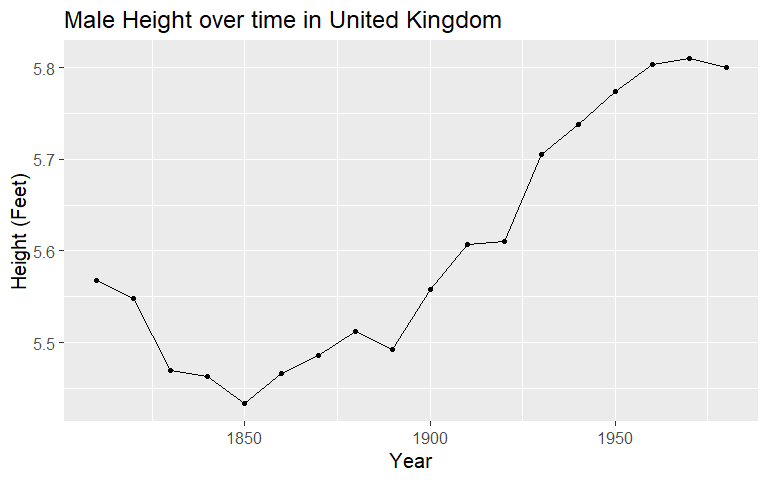# Lecture 6: Working with Data Part 2

## Recap

• We can get `data.frame`s by making them with `data.frame()`, or reading in data with `data()` or `read.csv`
• `data.frame`s are a list of vectors - we know vectors!
• We can pull the vectors back out with `\$`
• We can assign new variables, or update them, using `\$` as well

## Today

• We are going to continue working with `data.frame`s/`tibble`s
• And we’re going to introduce an important aspect of data analysis: splitting the data
• In other words, selecting only part of the data that we have
• In other words, to subset the data

## Why?

• Why would we want to do this?
• Many statistical questions require us to!
• We might be interested in how a variable differs for two different groups
• Or how one variable is related to another (i.e. how A looks for different values of B)
• Or how those relationships differ for different groups

## Example

• Let’s read in some data on male heights, from Our World in Data, and look at it
• Always look at the data before you use it!
• It has height in CM, let’s change that to feet
``````df <- read.csv('http://www.nickchk.com/average-height-men-OWID.csv')
str(df)``````
``````## 'data.frame':    1250 obs. of  4 variables:
##  \$ Entity  : Factor w/ 152 levels "Afghanistan",..: 1 1 1 2 2 2 3 3 3 4 ...
##  \$ Code    : Factor w/ 152 levels "AFG","AGO","ALB",..: 1 1 1 3 3 3 41 41 41 2 ...
##  \$ Year    : int  1870 1880 1930 1880 1890 1900 1910 1920 1930 1810 ...
##  \$ Heightcm: num  168 166 167 170 170 ...``````
``df <- df %>% mutate(Heightft = Heightcm/30.48)``

## Example

• If we look at height overall we will see that mean height is 167.64728
• But if we look at the data we can see that some countries aren’t present every year. So this isn’t exactly representative
``table(df\$Year)``
``````##
## 1810 1820 1830 1840 1850 1860 1870 1880 1890 1900 1910 1920 1930 1940 1950
##   35   28   29   41   49   59   72   80   82   86   80   85   91   72   91
## 1960 1970 1980
##   89   88   93``````
• So let’s just pick some countries that we DO have the full range of years on: the UK, Germany, France, the Congo, Gabon, and Nigeria!

## Example

• If we limit the data just to those three countries, we can see that the average height in these three, which covers 1810s-1980s evenly, is 168.8619048
• What if we want to compare the countries to each other? We need to split off each country by itself (let’s convert to feet, too).
``````## # A tibble: 6 x 2
##   Entity  Heightft
##   <chr>      <dbl>
## 1 Congo       5.44
## 2 France      5.52
## 3 Gabon       5.52
## 4 Germany     5.61
## 5 Nigeria     5.48
## 6 UK          5.60``````

## Example

• What questions does this answer?
• What is average height of men over this time period?
• How does average height differ across countries?
• What can’t we answer yet?
• How has height changed over time?
• What causes these height differences [later!]

## Example

• If we want to know how height changed over time, we need to evaluate each year separately too.## Example

• To compare the changes over time ACROSS countries, we evaluate separately by each year AND each country